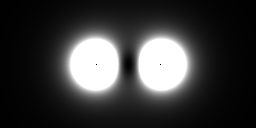# Field (physics) facts for kids

Kids Encyclopedia FactsThe magnitude of an electric field surrounding two equally charged (repelling) particles. Brighter areas have a greater magnitude. The direction of the field is not visible.

In physics, a field means that a physical quantity is assigned to every point in space (or, more generally, spacetime). A field is seen as extending throughout a large region of space so that it influences everything. The strength of a field usually varies over a region. Michael Faraday became the first to coin the term "field", in 1849.

For some fields, there is a number for each point in space. They are called scalar fields. For more complicated fields, there are more than one number for each point in space. They are called vector fields or tensor fields. For example, one can model a gravitational field by a vector field where a vector indicates the acceleration a mass would experience at each point in space. Other examples are temperature fields or air pressure fields, which are often illustrated on weather reports by isotherms and isobars by joining up the points of equal temperature or pressure respectively.

## Types of fields

### Classical fields

• Newtonian gravitation: describes the gravitational force as a mutual interaction between two masses.
• Electromagnetism: electric and magnetic fields are not only fields of force which dictate the motion of particles, but also have an independent physical reality because they carry energy.
• Gravitation in general relativity: this is Einstein's theory of gravity.
• Waves as fields

### Quantum fields

It is now believed that quantum mechanics should underlie all physical phenomena.

### Field theory

A field theory is a physical theory that describes how one or more physical fields interact with matter.

## Related pages

• Landau, Lev D. and Lifshitz, Evgeny M. 1971. Classical theory of fields. 3rd ed, London: Pergamon. Vol. 2 of the Course of Theoretical Physics.Field (physics) Facts for Kids. Kiddle Encyclopedia.